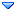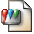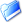GSI Forum
GSI Helmholtzzentrum für Schwerionenforschung

Home » PANDA » PandaRoot » Tracking » some options for eta_c analysis
some options for eta_c analysis Tue, 11 October 2011 14:55Dima Melnychuk Messages: 213Registered: April 2004 Location: National Centre for Nucle... first-grade participant From: *fuw.edu.pl
Hi,

I have tried several options in eta_c reconstruction as its was suggested by Stefano and here are the results.

I used for analysis data on grid run 925oldnocu (release july11).

1. Instead of selecting best eta_c candidate after vertex fit (using its chi2) I tried a preselection of best candidate using chi2 defined as (totalreco_mass-etac_mass)^2/sigma(eta_c)^2+(phi1reco_mass-phimass)^2/si gma(phi)+(phi2reco_mass-phimass)^2/sigma(phi).

And here there is a small improvement in efficiency and eta_c resolution and no improvement in phi mass resolution (efficiency 24.0%, sigma(eta_c)=32.8 MeV, sigma(phi)= 3.92 MeV).For comparison with selection of best candidate after vertex fit (efficiency 22.8%, sigma(eta_c)=33.2 MeV, sigma(phi)= 3.92 MeV).

2. I also tried to perform a mass fitter for phi mass and here is the eta_c mass resolution after combining 2 phi after this fitter (PndKinFitter).Here the best candidate is selected by difference between reconstructed and nominal eta_c mass and resolution is 34.7 MeV, i.e worse than with vertex fit. So I would exclude this option in eta_c analysis.

Dima
•Attachment: m_vertex_fit_preselection.png
•Attachment: m_vertex_fit.png
•Attachment: m_mass_fit.pngsome options for eta_c analysis By: Dima Melnychuk on Tue, 11 October 2011 14:55Re: some options for eta_c analysis By: StefanoSpataro on Tue, 11 October 2011 15:28